# Negative Frequency and Its Physical Meaning

Posted by in on 4-10-13

As electrical engineers, we’re called on for critical thinking on a constant basis. It’s often a good idea to step back a bit from the mathematics of your design to think about the physical meaning of the answers you’re getting to provide a very nice sanity check.

The catch is that concepts in electrical engineering are sometimes pretty difficult to visualize. For example, how do you visualize propagating waves interacting with an antenna surface? That’s pretty tough.

What about negative frequencies? Maybe you intuitively think about frequency as the rate of repetition. That’s not wrong, but it’s not the whole picture; a frequency spectrum has a negative and positive frequency axis. What could it possibly mean to have a negative repetition?

1. Physical Meaning of Negative Frequency
2. Deeper Explanation with Mathematics
3. Common Frequency Analysis Technique

## Physical Meaning of Negative Frequency

Remembering that physically, sinusoids are waves, the sign of the frequency represents the direction of wave propagation. Simply put, negative frequencies represent forward traveling waves, while positive frequencies represent backward traveling waves.

This sign relation is by convention. Electrical engineers define wave propagation as the following: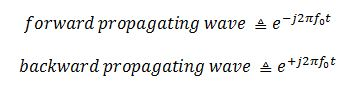It’s important to note that not all fields of science define wave propagation in the same sense. The most notable case is for physics. Physicists define wave propagation in exactly the opposite sense, with positive frequencies propagating forward.

The following two spectrums show the location of a forward-traveling and backward-traveling wave, respectively.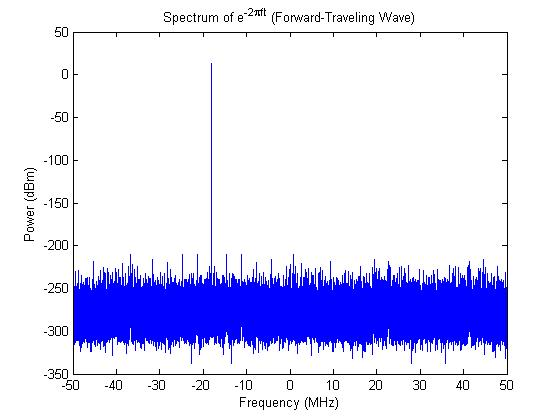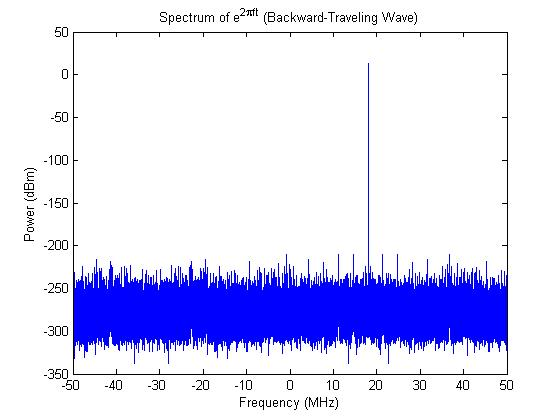Using a forward-propagating complex sinusoid as example, moving the sign from it’s typical location to in front of the frequency symbol illustrates the concept.## Deeper Explanation with Mathematics

Maybe you’re used to only modeling signals with trigonometric functions, like sine and cosine. So what happens when plot the spectrum of a cosine?You might be saying to yourself, “I didn’t specify a direction of propagation. It’s just a cosine!”. You actually did, and to prove it, let’s look at Euler’s equation: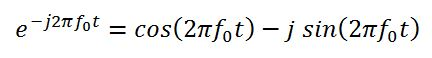Euler’s equation shows how a complex exponential is related to both the sine and cosine functions. The direction of propagation is much more obvious when looking at the complex form of sinusoids.

Keeping in mind the original cosine function that we plotted at the beginning of this section, let’s look at the cosine using Euler’s equation.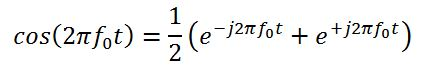Now it’s obvious that the cosine function splits the energy equally between forward and backward traveling waves!

## Common Frequency Analysis Technique

So what does it all mean, and how do I relate all this to real signals traveling down wires?

Whether or not you should care about positive and negative frequencies depends on how you got your time-domain data and your application.

If your samples are just real numbers, then you can ignore half the spectrum and double just one side. For real-sampled data, the spectrum is symmetrical, so it doesn’t matter which side you use. If your samples are complex (I and Q), you need both sides of the spectrum since the spectrum is asymmetric.

If you are modeling a system in MATLAB, decide whether or not you care about direction of propagation. For applications such as simple real-valued filters, you can just use cosines and look at a single-sided spectrum. If you’re modeling something where propagation and/or reflection matters (e.g. radar system), you should be modeling with complex exponentials. In that case you care about both sides of the spectrum.

For more information about the power spectrum and complex sampling, check out this post on the power spectrum.

In either method (real or complex data), energy is conserved; it’s all in how you look at it mathematically. Complex signals can be represented as sines and cosines; real signals can be represented with equivalent complex representations.

Look up how the Hilbert Transform works and is used. Keep your eyes peeled for a post on the Hilbert Transform in the future.

Special thanks to Santosh for suggesting this topic! You can request your own topic too by clicking the link at the top of the page.Did you enjoy this article?